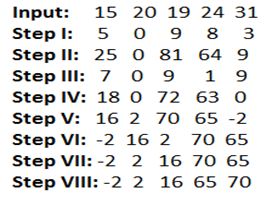# Machine Input Output Practice Set -Part:2

Directions (Q. 1-5): Study the following information carefully and answer the questions given below:
A number arrangement machine when given an input line of numbers rearranges them following a particular rule in each step. The following is an illustration of input and various steps of rearrangement.And Step VIII is the last step of the rearrangement as the desired arrangement is obtained. As per rules followed in the above steps, find out in each of the questions the appropriate step for the given input.
Input: 30 62 32 81 13 52

1. Which number is exactly between ‘63’ and ‘135’ in Step IV?
A. 27
B. 29
C. 0
D. 99
E. None of these

2. In which step the elements ‘135 27 63’ found in the same order?
A. III
B. II
C. V
D. IV
E. None of these

3. Which element comes exactly between '101' and '65' in step V of the given input?
A. 2
B. -2
C. 0
D. 25
E. None of these

4. If in the first step, "0" interchanges its position with '6' and '12' also interchanges its position with "8" then which element will be to the immediate left of '3'?
A. 20
B. 8
C. 12
D. 0
E. None of these

5. How many steps are needed to complete this arrangement?
A. VIII
B. VII
C. V
D. X
E. IX

1. A2. D

3. B

4. C

5. E